Chapter 10 Rotational Motion and Angular Momentum

# 10.5 Angular Momentum and Its Conservation

### Summary

• Understand the analogy between angular momentum and linear momentum.
• Observe the relationship between torque and angular momentum.
• Apply the law of conservation of angular momentum.

Why does Earth keep on spinning? What started it spinning to begin with? And how does an ice skater manage to spin faster and faster simply by pulling her arms in? Why does she not have to exert a torque to spin faster? Questions like these have answers based in angular momentum, the rotational analog to linear momentum.

By now the pattern is clear—every rotational phenomenon has a direct translational analog. It seems quite reasonable, then, to define angular momentum L as

$\boldsymbol{L=I\omega}.$

This equation is an analog to the definition of linear momentum as p=mv. Units for linear momentum are kg⋅m/s while units for angular momentum are kg⋅m2/s. As we would expect, an object that has a large moment of inertia I, such as Earth, has a very large angular momentum. An object that has a large angular velocity ω, such as a centrifuge, also has a rather large angular momentum.

### MAKING CONNECTIONS

Angular momentum is completely analogous to linear momentum, first presented in Chapter 6 Uniform Circular Motion and Gravitation. It has the same implications in terms of carrying rotation forward, and it is conserved when the net external torque is zero. Angular momentum, like linear momentum, is also a property of the atoms and subatomic particles.

### Example 1: Calculating Angular Momentum of the Earth

Strategy

No information is given in the statement of the problem; so we must look up pertinent data before we can calculate L=Iω. First, according to Chapter 10.3 Making Connections, the formula for the moment of inertia of a sphere is

$\boldsymbol{I\:=}$$\boldsymbol{\frac{2MR^2}{5}}$

so that

$\boldsymbol{L=I\omega=}$$\boldsymbol{\frac{2MR^2\omega}{5}}.$

Earth’s mass M is 5.979 × 1024 kg and its radius R is 6.376 × 106 m.The Earth’s angular velocity ω is, of course, exactly one revolution per day, but we must covert ω to radians per second to do the calculation in SI units.

Solution

Substituting known information into the expression for L and converting ω to radians per second gives

$\begin{array}{lcl} \boldsymbol{L} & \boldsymbol{=} & \boldsymbol{0.4(5.979\times10^{24}\textbf{ kg})(6.376\times10^6\textbf{ m})^2(\frac{1\textbf{ rev}}{d})} \\ {} & \boldsymbol{=} & \boldsymbol{9.72\times10^{37}\textbf{ kg}\cdotp\textbf{m}^2\cdotp\textbf{rev/d.}} \end{array}$

Substituting rad for 1 rev and 8.64 × 104 s for 1 day gives

$\begin{array}{lcl} \boldsymbol{L} & \boldsymbol{=} & \boldsymbol{(9.72\times10^{37}\textbf{ kg}\cdotp\textbf{m}^2)(\frac{2\pi\textbf{ rad/rev}}{8.64\times10^4\textbf{ s/d}})(1\textbf{ rev/d})} \\ {} & \boldsymbol{=} & \boldsymbol{7.07\times10^{33}\textbf{ kg}\cdotp\textbf{m}^2\textbf{/s.}} \end{array}$

Discussion

This number is large, demonstrating that Earth, as expected, has a tremendous angular momentum. The answer is approximate, because we have assumed a constant density for Earth in order to estimate its moment of inertia.

When you push a merry-go-round, spin a bike wheel, or open a door, you exert a torque. If the torque you exert is greater than opposing torques, then the rotation accelerates, and angular momentum increases. The greater the net torque, the more rapid the increase in L. The relationship between torque and angular momentum is

$\boldsymbol{\textbf{net }\tau\:=}$$\boldsymbol{\frac{\Delta{L}}{\Delta{t}}.}$

This expression is exactly analogous to the relationship between force and linear momentum, Fpt. The equation $\boldsymbol{\textbf{net }\tau=\frac{\Delta{L}}{\Delta{t}}}$ is very fundamental and broadly applicable. It is, in fact, the rotational form of Newton’s second law.

### Example 2: Calculating the Torque Putting Angular Momentum Into a Lazy Susan

Figure 1 shows a Lazy Susan food tray being rotated by a person in quest of sustenance. Suppose the person exerts a 2.50 N force perpendicular to the lazy Susan’s 0.260-m radius for 0.150 s. (a) What is the final angular momentum of the lazy Susan if it starts from rest, assuming friction is negligible? (b) What is the final angular velocity of the lazy Susan, given that its mass is 4.00 kg and assuming its moment of inertia is that of a disk?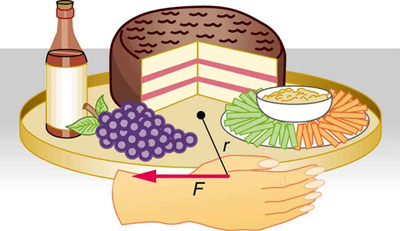Figure 1. A partygoer exerts a torque on a lazy Susan to make it rotate. The equation net τ=ΔL/Δt gives the relationship between torque and the angular momentum produced.

Strategy

We can find the angular momentum by solving $\boldsymbol{\textbf{net }\tau=\frac{\Delta{L}}{\Delta{t}}}$ for ΔL, and using the given information to calculate the torque. The final angular momentum equals the change in angular momentum, because the lazy Susan starts from rest. That is, ΔL=L. To find the final velocity, we must calculate ω from the definition of L in L=Iω.

Solution for (a)

Solving $\boldsymbol{\textbf{net }\tau=\frac{\Delta{L}}{\Delta{t}}}$ for ΔL gives

$\boldsymbol{\Delta{L}=\textbf{net }\tau\Delta{t}}.$

Because the force is perpendicular to r, we see that net τ=rF, so that

$\begin{array}{lcl} \boldsymbol{L} & \boldsymbol{=} & \boldsymbol{\textbf{rF}\Delta{t}=(0.260\textbf{ m})(2.50\textbf{ N})(0.150\textbf{ s})} \\ {} & \boldsymbol{=} & \boldsymbol{9.75\times10^{-2}\textbf{ kg}\cdotp\textbf{m}^2\textbf{/s.}} \end{array}$

Solution for (b)

The final angular velocity can be calculated from the definition of angular momentum,

$\boldsymbol{L=I\omega.}$

Solving for ω and substituting the formula for the moment of inertia of a disk into the resulting equation gives

$\boldsymbol{\omega\:=}$$\boldsymbol{\frac{L}{I}}$$\boldsymbol{=}$$\boldsymbol{\frac{L}{\frac{1}{2}MR^2}}.$

And substituting known values into the preceding equation yields

$\boldsymbol{\omega\:=}$$\boldsymbol{\frac{9.75\times10^{-2}\textbf{ kg}\cdot\textbf{m}^2\textbf{/s}}{(0.500)(4.00\textbf{ kg})(0.260\textbf{ m})}}$$\boldsymbol{=0.721\textbf{ rad/s.}}$

Discussion

Note that the imparted angular momentum does not depend on any property of the object but only on torque and time. The final angular velocity is equivalent to one revolution in 8.71 s (determination of the time period is left as an exercise for the reader), which is about right for a lazy Susan.

### Example 3: Calculating the Torque in a Kick

The person whose leg is shown in Figure 2 kicks his leg by exerting a 2000-N force with his upper leg muscle. The effective perpendicular lever arm is 2.20 cm. Given the moment of inertia of the lower leg is 1.25 kg⋅m2, (a) find the angular acceleration of the leg. (b) Neglecting the gravitational force, what is the rotational kinetic energy of the leg after it has rotated through 57.3° (1.00 rad)?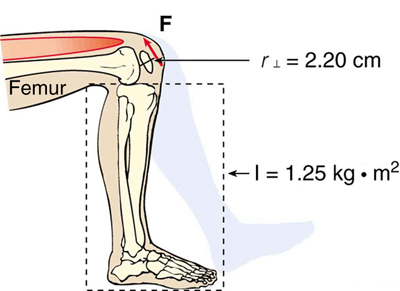Figure 2. The muscle in the upper leg gives the lower leg an angular acceleration and imparts rotational kinetic energy to it by exerting a torque about the knee. F is a vector that is perpendicular to r. This example examines the situation.

Strategy

The angular acceleration can be found using the rotational analog to Newton’s second law, or $\boldsymbol{\alpha=\textbf{net }\tau/I}.$ The moment of inertia I is given and the torque can be found easily from the given force and perpendicular lever arm. Once the angular acceleration $\boldsymbol{\alpha}$ is known, the final angular velocity and rotational kinetic energy can be calculated.

Solution to (a)

From the rotational analog to Newton’s second law, the angular acceleration $\boldsymbol{\alpha}$ is

$\boldsymbol{\alpha=}$$\boldsymbol{\frac{\textbf{net}\;\tau}{{I}}}.$

Because the force and the perpendicular lever arm are given and the leg is vertical so that its weight does not create a torque, the net torque is thus

$\begin{array}{lcl} \boldsymbol{\textbf{net }\tau} & \boldsymbol{=} & \boldsymbol{r_{\perp}F} \\ {} & \boldsymbol{=} & \boldsymbol{(0.0220\textbf{ m})(2000\textbf{ N})} \\ {} & \boldsymbol{=} & \boldsymbol{44.0\textbf{ N}\cdotp\textbf{m.}} \end{array}$

Substituting this value for the torque and the given value for the moment of inertia into the expression for $\boldsymbol{\alpha}$ gives

$\boldsymbol{\alpha\:=}$$\boldsymbol{\frac{44.0\textbf{ N}\cdotp\textbf{m}}{1.25\textbf{ kg}\cdotp\textbf{m}^2}}$$\boldsymbol{=\:35.2\textbf{ rad/s}^2}.$

Solution to (b)

The final angular velocity can be calculated from the kinematic expression

$\boldsymbol{\omega^2=\omega_0^2+2\alpha\theta}$

or

$\boldsymbol{\omega^2=2\alpha\theta}$

because the initial angular velocity is zero. The kinetic energy of rotation is

$\boldsymbol{\textbf{KE}_{\textbf{rot}}\:=}$$\boldsymbol{\frac{1}{2}}$$\boldsymbol{I\omega^2}$

so it is most convenient to use the value of ω2 just found and the given value for the moment of inertia. The kinetic energy is then

$\begin{array}{lcl} \textbf{KE}_{\textbf{rot}} & \boldsymbol{=} & \boldsymbol{0.5(1.25\textbf{ kg}\cdotp\textbf{m}^2)(70.4\textbf{ rad}^2\textbf{/s}^2)} \\ {} & \boldsymbol{=} & \boldsymbol{44.0\textbf{ J.}} \end{array}$

Discussion

These values are reasonable for a person kicking his leg starting from the position shown. The weight of the leg can be neglected in part (a) because it exerts no torque when the center of gravity of the lower leg is directly beneath the pivot in the knee. In part (b), the force exerted by the upper leg is so large that its torque is much greater than that created by the weight of the lower leg as it rotates. The rotational kinetic energy given to the lower leg is enough that it could give a ball a significant velocity by transferring some of this energy in a kick.

### MAKING CONNECTIONS: CONSERVATION LAWS

Angular momentum, like energy and linear momentum, is conserved. This universally applicable law is another sign of underlying unity in physical laws. Angular momentum is conserved when net external torque is zero, just as linear momentum is conserved when the net external force is zero.

# Conservation of Angular Momentum

We can now understand why Earth keeps on spinning. As we saw in the previous example, ΔL=(net τ)Δt. This equation means that, to change angular momentum, a torque must act over some period of time. Because Earth has a large angular momentum, a large torque acting over a long time is needed to change its rate of spin. So what external torques are there? Tidal friction exerts torque that is slowing Earth’s rotation, but tens of millions of years must pass before the change is very significant. Recent research indicates the length of the day was 18 h some 900 million years ago. Only the tides exert significant retarding torques on Earth, and so it will continue to spin, although ever more slowly, for many billions of years.

What we have here is, in fact, another conservation law. If the net torque is zero, then angular momentum is constant or conserved. We can see this rigorously by considering $\boldsymbol{\textbf{net }\tau=\frac{\Delta{L}}{\Delta{t}}}$ for the situation in which the net torque is zero. In that case,

$\boldsymbol{\textbf{net}\tau=0}$

implying that

$\boldsymbol{\frac{\Delta{L}}{\Delta{t}}}$$\boldsymbol{=0}.$

If the change in angular momentum ΔL is zero, then the angular momentum is constant; thus,

$\boldsymbol{L=\textbf{constant }(\textbf{net }\tau=0)}$

or

$\boldsymbol{L=L^{\prime}(\textbf{net }\tau=0).}$

These expressions are the law of conservation of angular momentum. Conservation laws are as scarce as they are important.

An example of conservation of angular momentum is seen in Figure 3, in which an ice skater is executing a spin. The net torque on her is very close to zero, because there is relatively little friction between her skates and the ice and because the friction is exerted very close to the pivot point. (Both F and r are small, and so τ is negligibly small.) Consequently, she can spin for quite some time. She can do something else, too. She can increase her rate of spin by pulling her arms and legs in. Why does pulling her arms and legs in increase her rate of spin? The answer is that her angular momentum is constant, so that

$\boldsymbol{L=L^{\prime}.}$

Expressing this equation in terms of the moment of inertia,

$\boldsymbol{I\omega=I^{\prime}\omega^{\prime},}$

where the primed quantities refer to conditions after she has pulled in her arms and reduced her moment of inertia. Because I is smaller, the angular velocity ω′ must increase to keep the angular momentum constant. The change can be dramatic, as the following example shows.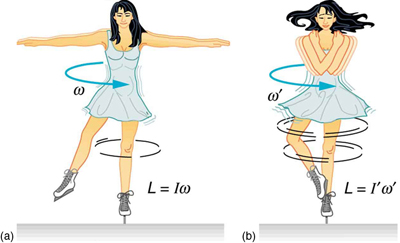Figure 3. (a) An ice skater is spinning on the tip of her skate with her arms extended. Her angular momentum is conserved because the net torque on her is negligibly small. In the next image, her rate of spin increases greatly when she pulls in her arms, decreasing her moment of inertia. The work she does to pull in her arms results in an increase in rotational kinetic energy.

### Example 4: Calculating the Angular Momentum of a Spinning Skater

Suppose an ice skater, such as the one in Figure 3, is spinning at 0.800 rev/ s with her arms extended. She has a moment of inertia of 2.34 kg⋅m2 with her arms extended and of 0.363 kg⋅m2 with her arms close to her body. (These moments of inertia are based on reasonable assumptions about a 60.0-kg skater.) (a) What is her angular velocity in revolutions per second after she pulls in her arms? (b) What is her rotational kinetic energy before and after she does this?

Strategy

In the first part of the problem, we are looking for the skater’s angular velocity ω′ after she has pulled in her arms. To find this quantity, we use the conservation of angular momentum and note that the moments of inertia and initial angular velocity are given. To find the initial and final kinetic energies, we use the definition of rotational kinetic energy given by

$\boldsymbol{\textbf{KE}_{\textbf{rot}}\:=}$$\boldsymbol{\frac{1}{2}}$$\boldsymbol{I\omega^2.}$

Solution for (a)

Because torque is negligible (as discussed above), the conservation of angular momentum given in Iω=I′ω′ is applicable. Thus,

$\boldsymbol{L=L^{\prime}}$

or

$\boldsymbol{I\omega=I^{\prime}\omega^{\prime}}$

Solving for ω′ and substituting known values into the resulting equation gives

$\begin{array}{lcl} \boldsymbol{\omega^{\prime}} & \boldsymbol{=} & \boldsymbol{\frac{I}{I^{\prime}}\omega=(\frac{2.34\textbf{ kg}\cdotp\textbf{m}^2}{0.363\textbf{ kg}\cdotp\textbf{m}^2})(0.800\textbf{ rev/s})} \\ {} & \boldsymbol{=} & \boldsymbol{5.16\textbf{ rev/s.}} \end{array}$

Solution for (b)

Rotational kinetic energy is given by

$\boldsymbol{\textbf{KE}_{\textbf{rot}}\:=}$$\boldsymbol{\frac{1}{2}}$$\boldsymbol{I\omega^2.}$

The initial value is found by substituting known values into the equation and converting the angular velocity to rad/s:

$\begin{array}{lcl} \textbf{KE}_{\textbf{rot}} & \boldsymbol{=} & \boldsymbol{(0.5)(2.34\textbf{ kg}\cdotp\textbf{m}^2)((0.800\textbf{ rev/s})(2\pi\textbf{ rad/rev}))^2} \\ {} & \boldsymbol{=} & \boldsymbol{29.6\textbf{ J.}} \end{array}$

The final rotational kinetic energy is

$\boldsymbol{\textbf{KE}_{\textbf{rot}}^{\prime}\:=}$$\boldsymbol{\frac{1}{2}}$$\boldsymbol{I^{\prime}{\omega}^{\prime{2}}}$

Substituting known values into this equation gives

$\begin{array}{lcl} \boldsymbol{\textbf{KE}_{\textbf{rot}}^{\prime}} & \boldsymbol{=} & \boldsymbol{(0.5)(0.363\textbf{ kg}\cdotp\textbf{m}^2)[(5.16\textbf{ rev/s})(2\pi\textbf{ rad/rev})]^2} \\ {} & \boldsymbol{=} & \boldsymbol{191\textbf{ J.}} \end{array}$

Discussion

In both parts, there is an impressive increase. First, the final angular velocity is large, although most world-class skaters can achieve spin rates about this great. Second, the final kinetic energy is much greater than the initial kinetic energy. The increase in rotational kinetic energy comes from work done by the skater in pulling in her arms. This work is internal work that depletes some of the skater’s food energy.

There are several other examples of objects that increase their rate of spin because something reduced their moment of inertia. Tornadoes are one example. Storm systems that create tornadoes are slowly rotating. When the radius of rotation narrows, even in a local region, angular velocity increases, sometimes to the furious level of a tornado. Earth is another example. Our planet was born from a huge cloud of gas and dust, the rotation of which came from turbulence in an even larger cloud. Gravitational forces caused the cloud to contract, and the rotation rate increased as a result. (See Figure 4.)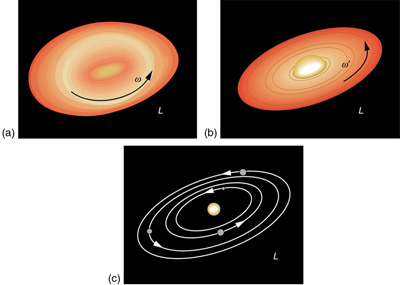Figure 4. The Solar System coalesced from a cloud of gas and dust that was originally rotating. The orbital motions and spins of the planets are in the same direction as the original spin and conserve the angular momentum of the parent cloud.

In case of human motion, one would not expect angular momentum to be conserved when a body interacts with the environment as its foot pushes off the ground. Astronauts floating in space aboard the International Space Station have no angular momentum relative to the inside of the ship if they are motionless. Their bodies will continue to have this zero value no matter how they twist about as long as they do not give themselves a push off the side of the vessel.

1: Is angular momentum completely analogous to linear momentum? What, if any, are their differences?

# Section Summary

• Every rotational phenomenon has a direct translational analog , likewise angular momentum L can be defined as L=Iω.
• This equation is an analog to the definition of linear momentum as p=mv. The relationship between torque and angular momentum is $\boldsymbol{\textbf{net }\tau=\frac{\Delta{L}}{\Delta{t}}}.$
• Angular momentum, like energy and linear momentum, is conserved. This universally applicable law is another sign of underlying unity in physical laws. Angular momentum is conserved when net external torque is zero, just as linear momentum is conserved when the net external force is zero.

### Conceptual Questions

1: When you start the engine of your car with the transmission in neutral, you notice that the car rocks in the opposite sense of the engine’s rotation. Explain in terms of conservation of angular momentum. Is the angular momentum of the car conserved for long (for more than a few seconds)?

2: Suppose a child walks from the outer edge of a rotating merry-go round to the inside. Does the angular velocity of the merry-go-round increase, decrease, or remain the same? Explain your answer.

3: Suppose a child gets off a rotating merry-go-round. Does the angular velocity of the merry-go-round increase, decrease, or remain the same if: (a) He jumps off radially? (b) He jumps backward to land motionless? (c) He jumps straight up and hangs onto an overhead tree branch? (d) He jumps off forward, tangential to the edge? Explain your answers. (Refer to Figure 5).

4: Helicopters have a small propeller on their tail to keep them from rotating in the opposite direction of their main lifting blades. Explain in terms of Newton’s third law why the helicopter body rotates in the opposite direction to the blades.

5: Whenever a helicopter has two sets of lifting blades, they rotate in opposite directions (and there will be no tail propeller). Explain why it is best to have the blades rotate in opposite directions.

6: Describe how work is done by a skater pulling in her arms during a spin. In particular, identify the force she exerts on each arm to pull it in and the distance each moves, noting that a component of the force is in the direction moved. Why is angular momentum not increased by this action?

7: When there is a global heating trend on Earth, the atmosphere expands and the length of the day increases very slightly. Explain why the length of a day increases.

8: Nearly all conventional piston engines have flywheels on them to smooth out engine vibrations caused by the thrust of individual piston firings. Why does the flywheel have this effect?

9: Jet turbines spin rapidly. They are designed to fly apart if something makes them seize suddenly, rather than transfer angular momentum to the plane’s wing, possibly tearing it off. Explain how flying apart conserves angular momentum without transferring it to the wing.

10: An astronaut tightens a bolt on a satellite in orbit. He rotates in a direction opposite to that of the bolt, and the satellite rotates in the same direction as the bolt. Explain why. If a handhold is available on the satellite, can this counter-rotation be prevented? Explain your answer.

11: Competitive divers pull their limbs in and curl up their bodies when they do flips. Just before entering the water, they fully extend their limbs to enter straight down. Explain the effect of both actions on their angular velocities. Also explain the effect on their angular momenta.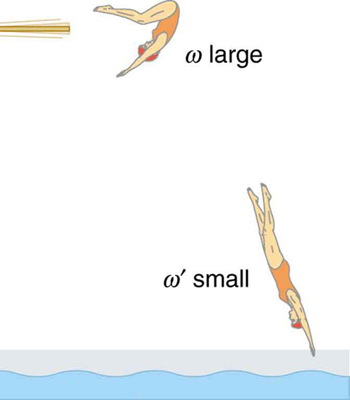Figure 6. The diver spins rapidly when curled up and slows when she extends her limbs before entering the water.

12: Draw a free body diagram to show how a diver gains angular momentum when leaving the diving board.

13: In terms of angular momentum, what is the advantage of giving a football or a rifle bullet a spin when throwing or releasing it?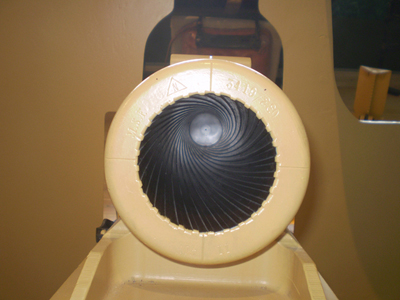Figure 7. The image shows a view down the barrel of a cannon, emphasizing its rifling. Rifling in the barrel of a canon causes the projectile to spin just as is the case for rifles (hence the name for the grooves in the barrel). (credit: Elsie esq., Flickr)

### Problems & Exercises

1: (a) Calculate the angular momentum of the Earth in its orbit around the Sun.

(b) Compare this angular momentum with the angular momentum of Earth on its axis.

2: (a) What is the angular momentum of the Moon in its orbit around Earth?

(b) How does this angular momentum compare with the angular momentum of the Moon on its axis? Remember that the Moon keeps one side toward Earth at all times.

(c) Discuss whether the values found in parts (a) and (b) seem consistent with the fact that tidal effects with Earth have caused the Moon to rotate with one side always facing Earth.

3: Suppose you start an antique car by exerting a force of 300 N on its crank for 0.250 s. What angular momentum is given to the engine if the handle of the crank is 0.300 m from the pivot and the force is exerted to create maximum torque the entire time?

4: A playground merry-go-round has a mass of 120 kg and a radius of 1.80 m and it is rotating with an angular velocity of 0.500 rev/s. What is its angular velocity after a 22.0-kg child gets onto it by grabbing its outer edge? The child is initially at rest.

5: Three children are riding on the edge of a merry-go-round that is 100 kg, has a 1.60-m radius, and is spinning at 20.0 rpm. The children have masses of 22.0, 28.0, and 33.0 kg. If the child who has a mass of 28.0 kg moves to the center of the merry-go-round, what is the new angular velocity in rpm?

6: (a) Calculate the angular momentum of an ice skater spinning at 6.00 rev/s given his moment of inertia is 0.400 kg⋅m2. (b) He reduces his rate of spin (his angular velocity) by extending his arms and increasing his moment of inertia. Find the value of his moment of inertia if his angular velocity decreases to 1.25 rev/s. (c) Suppose instead he keeps his arms in and allows friction of the ice to slow him to 3.00 rev/s. What average torque was exerted if this takes 15.0 s?

Consider the Earth-Moon system. Construct a problem in which you calculate the total angular momentum of the system including the spins of the Earth and the Moon on their axes and the orbital angular momentum of the Earth-Moon system in its nearly monthly rotation. Calculate what happens to the Moon’s orbital radius if the Earth’s rotation decreases due to tidal drag. Among the things to be considered are the amount by which the Earth’s rotation slows and the fact that the Moon will continue to have one side always facing the Earth.

## Glossary

angular momentum
the product of moment of inertia and angular velocity
law of conservation of angular momentum
angular momentum is conserved, i.e., the initial angular momentum is equal to the final angular momentum when no external torque is applied to the system

### Solutions

1: Yes, angular and linear momentums are completely analogous. While they are exact analogs they have different units and are not directly inter-convertible like forms of energy are.

Problems & Exercises

1: (a) $\boldsymbol{2.66\times10^{40}\textbf{ kg}\cdotp\textbf{m}^2\textbf{/s}}$ (b) $\boldsymbol{7.07\times10^{33}\textbf{ kg}\cdotp\textbf{m}^2\textbf{/s}}$

The angular momentum of the Earth in its orbit around the Sun is $\boldsymbol{3.77\times10^6}$ times larger than the angular momentum of the Earth around its axis.

3: $\boldsymbol{22.5\textbf{ kg}\cdotp\textbf{m}^2\textbf{/s}}$

5: $$\boldsymbol{25.3\textbf{ rpm}}$$